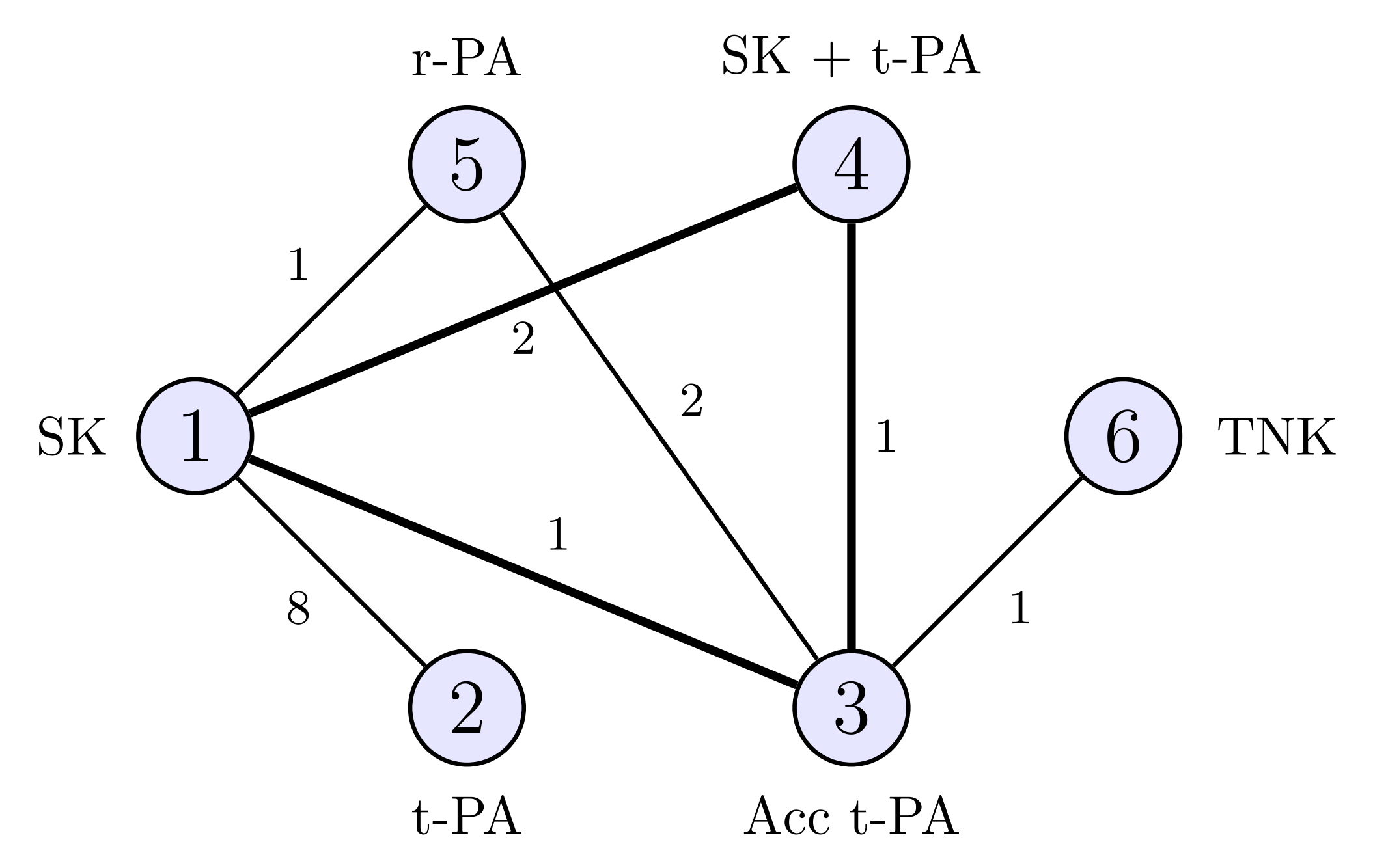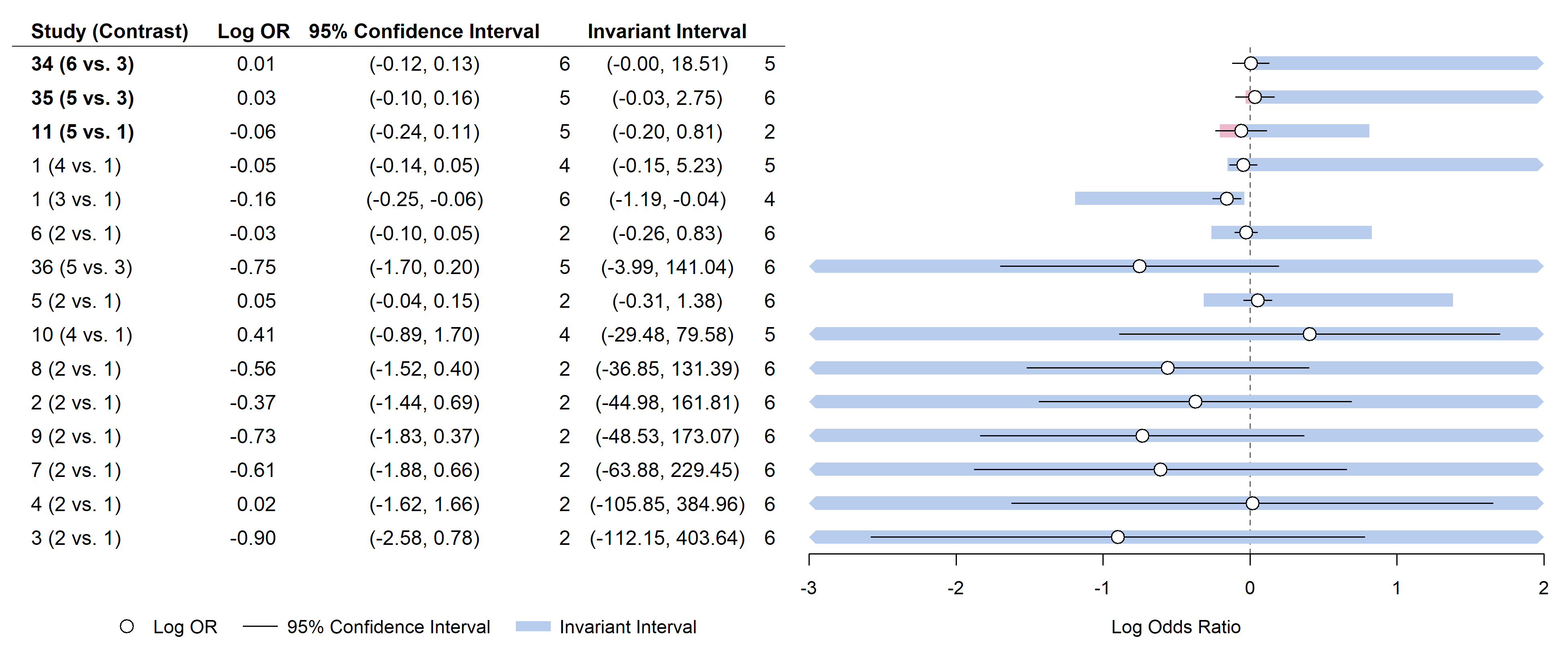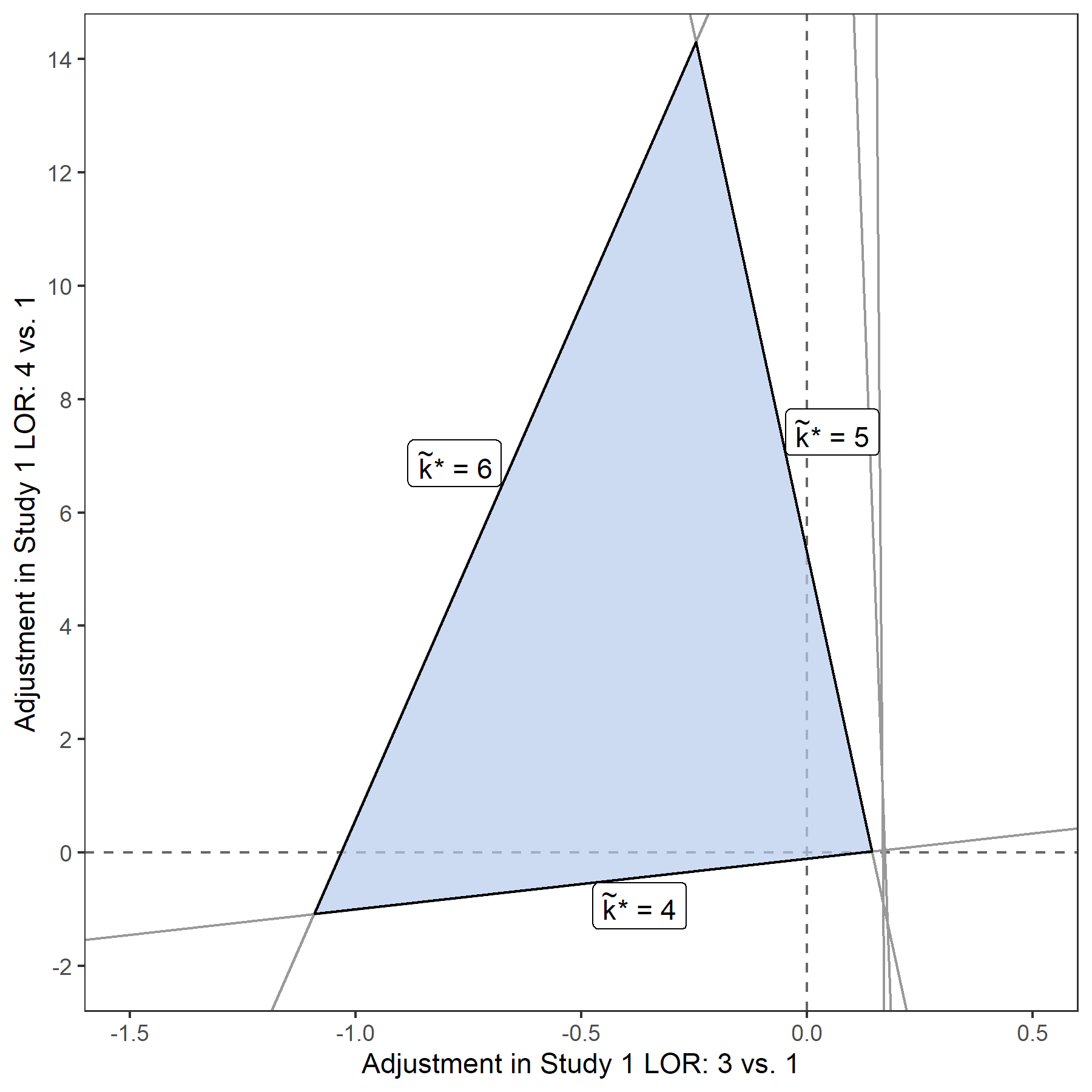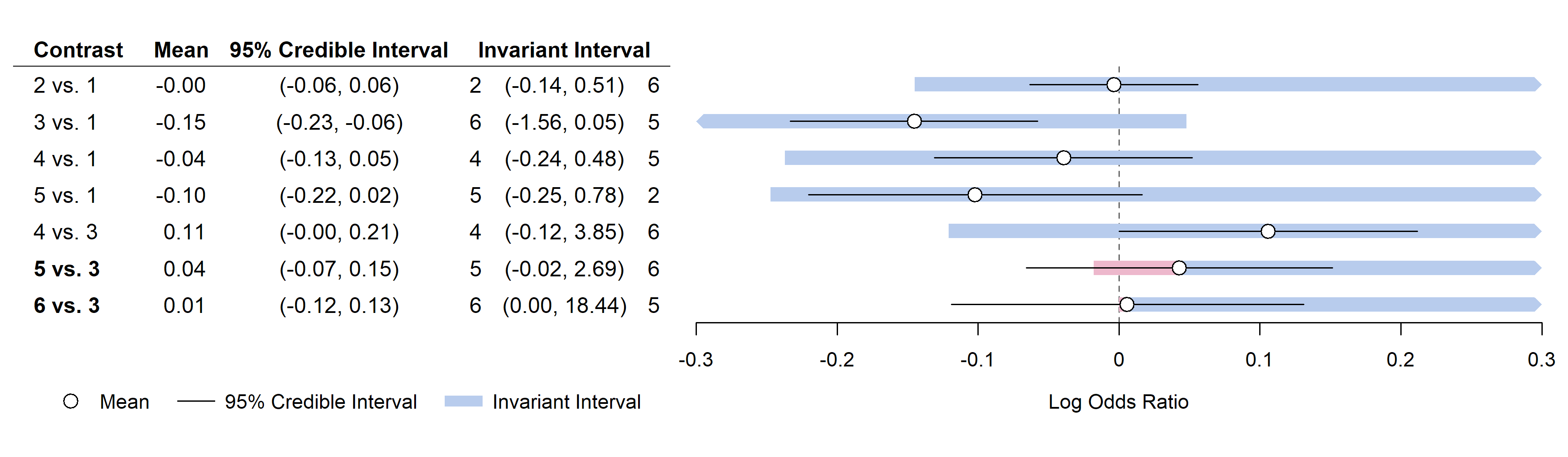# Introduction

This vignette provides worked examples for the `nmathresh` package, recreating exactly the analyses in the paper by Phillippo et al. (2018).

# Thrombolytics

Caldwell et al. (2005) present a NMA of six thrombolytic treatments, with data taken from two systematic reviews (Boland et al. 2003, Keeley et al. 2003).Thrombolytic treatment network, from Phillippo et al. (2018).

The results of the NMA are available in the `nmathresh` package, as `Thrombo.post.summary` (for the posterior summaries) and `Thrombo.post.cov` (for the posterior covariance matrix). The posterior summaries were generated using the `coda` package from saved WinBUGS chains and are stored as `summary.mcmc` objects, but the `coda` package is not required for our analysis.

``````library(nmathresh)

# library(coda)   # Not required - but prints the summary in a nicer format
# Thrombo.post.summary``````

## Study level threshold analysis

To run a study level threshold analysis, we require the study data. This is available in `nmathresh` as a tab-delimited text file, and is read in like so:

``````dat.raww <- read.delim(system.file("extdata", "Thrombo_data.txt", package = "nmathresh"))

# print first few rows
``````##    r.1   n.1  r.2   n.2 r.3   n.3 t.1 t.2 t.3 n_arms studyID
## 1 1472 20251  652 10396 723 10374   1   3   4      3       1
## 2    9   130    6   123  NA    NA   1   2  NA      2       2
## 3    5    63    2    59  NA    NA   1   2  NA      2       3
## 4    3    65    3    64  NA    NA   1   2  NA      2       4
## 5  887 10396  929 10372  NA    NA   1   2  NA      2       5
## 6 1455 13780 1418 13746  NA    NA   1   2  NA      2       6``````
``n <- nrow(dat.raww)   # number of studies``

Thresholds will be derived on the log odds ratio scale, so we derive log odds ratios against arm 1 as a reference.

``````# Log OR for two-arm trials, arm 2 vs. 1
dat.raww\$lor.1 <- with(dat.raww, log(r.2 * (n.1 - r.1) / ((n.2 - r.2) * r.1)) )

dat.raww\$k.1 <- dat.raww\$t.2   # Arm 2 treatment
dat.raww\$b.1 <- dat.raww\$t.1   # Reference treatment

# Log OR for three-arm trials, arm 3 vs. 1
dat.raww\$lor.2 <- with(dat.raww, log(r.3 * (n.1 - r.1) / ((n.3 - r.3) * r.1)) )

dat.raww\$k.2 <- dat.raww\$t.3   # Arm 3 treatment (NA if only 2 arms)
dat.raww\$b.2 <- ifelse(is.na(dat.raww\$k.2), NA, dat.raww\$t.1)   # Reference treatment``````

The likelihood covariance matrix is then constructed in a block diagonal manner, with the aid of `Matrix::bdiag`.

``````# LOR variances and covariances, likelihood covariance matrix V
V.diag <- as.list(rep(NA, n))
attach(dat.raww)
for (i in 1:n){
if (dat.raww\$n_arms[i] == 2){
V.diag[[i]] <- 1/r.1[i] + 1/r.2[i] + 1/(n.1[i]-r.1[i]) + 1/(n.2[i]-r.2[i])
}
else if (dat.raww\$n_arms[i] == 3){
v1 <- 1/r.1[i] + 1/r.2[i] + 1/(n.1[i]-r.1[i]) + 1/(n.2[i]-r.2[i])
v2 <- 1/r.1[i] + 1/r.3[i] + 1/(n.1[i]-r.1[i]) + 1/(n.3[i]-r.3[i])
# Covariance term
c1 <- 1/r.1[i] + 1/(n.1[i] - r.1[i])
V.diag[[i]] <- matrix(c(v1, c1, c1, v2), nrow = 2)
}
}
detach(dat.raww)

library(Matrix)
V <- bdiag(V.diag)``````

The raw data was imported in wide format, with one row per study. It is much easier to work with the data in long format, with one row per data point (contrast).

``````# Reshape the data to have one row per contrast per study
dat.rawl <- reshape(dat.raww, varying = c("lor.1", "b.1", "k.1", "lor.2", "b.2", "k.2"),
timevar = "c", idvar = "studyID", direction = "long")

# Sort data by study and contrast, removing NA rows
dat.rawl <- dat.rawl[order(dat.rawl\$studyID, dat.rawl\$c, dat.rawl\$b, na.last = NA), ]

N <- nrow(dat.rawl)   # number of data points
K <- length(unique(c(dat.rawl\$b, dat.rawl\$k)))   # Number of treatments``````

Construct the design matrix.

``````# Construct the design matrix, with a row for each contrast and K-1 columns (parameters)
X <- matrix(0, nrow = N, ncol = K-1)

for (i in 1:N){
X[i, dat.rawl\$k[i]-1] <- 1
if (dat.rawl\$b[i] != 1){
X[i, dat.rawl\$b[i]-1] <- -1
}
}``````

We are now ready to perform a threshold analysis at the study level. This is made easy using the `nma_thresh` function, which takes the posterior means and covariance matrix of the treatment effect parameters (\(d_k\)), the likelihood covariance matrix, and the design matrix. We specify `nmatype = "fixed"` to derive thresholds for the FE model, and `opt.max = FALSE` since the optimal treatment is the one which minimises the log odds.

``````# Now we can perform thresholding at the study level
thresh <- nma_thresh(mean.dk = Thrombo.post.summary\$statistics[1:(K-1), "Mean"],
lhood = V,
post = Thrombo.post.cov,
nmatype = "fixed",
X = X,
opt.max = FALSE)``````
``## Likelihood for N = 15 data points.``
``## Number of treatments is K = 6.``
``## Current optimal treatment is k* = 3.``

The `nma_thresh` function prints some basic details, which can be used to verify that the input was as expected (the number of data points and treatments, and the base-case optimal treatment). These are also shown when the threshold object is printed:

``thresh``
``````## A thresh object. For help, see ?'thresh-class'.
##
## Base-case optimal treatment is k* = 3.
##
##             lo lo.newkstar          hi hi.newkstar
## 1   -1.0318603           6   0.1197831           4
## 2   -0.1071759           4   5.2771792           5
## 3  -44.6044047           2 162.1861914           6
## 4 -111.2554454           2 404.5362135           6
## 5 -105.8657622           2 384.9387723           6
## 6   -0.3656429           2   1.3295151           6
## ... 9 further rows omitted ...``````

Finally, we will use the function `thresh_forest` to display the thresholds on a forest plot. We sort the rows of the plot to display those with smallest thresholds first; this is achieved using the `orderby` option. (By default, `thresh_forest` calculates recommended output dimensions, which are useful for saving to PDF. This isn’t necessary here, so we set `calcdim = FALSE`.)

``````# Display using a forest plot, along with 95% confidence intervals for LORs
# Create row labels
dat.rawl\$lab <- rep(NA, nrow(dat.rawl))
for (i in 1:nrow(dat.rawl)) {
dat.rawl\$lab[i] <- paste0(dat.rawl\$studyID[i], " (", dat.rawl\$k[i], " vs. ", dat.rawl\$b[i], ")")
}

# Calculate 95% CIs
dat.rawl\$CI2.5 <- dat.rawl\$lor + qnorm(.025)*sqrt(diag(V))
dat.rawl\$CI97.5 <- dat.rawl\$lor + qnorm(.975)*sqrt(diag(V))

# Calculate the proportion of CI covered by invariant interval, for sorting.
# Coverage <1 means that the CI extends beyond the bias invariant threshold, and
# the threshold is below the level of statistical uncertainty.
dat.rawl\$coverage <- apply(cbind(thresh\$thresholds\$lo / (dat.rawl\$CI2.5 - dat.rawl\$lor),
thresh\$thresholds\$hi / (dat.rawl\$CI97.5 - dat.rawl\$lor)),
1, min, na.rm = TRUE)

# Plot
thresh_forest(thresh,
y = lor, CI.lo = CI2.5, CI.hi = CI97.5,
label = lab, orderby = coverage, data = dat.rawl,
CI.title = "95% Confidence Interval", y.title = "Log OR",
label.title = "Study (Contrast)", xlab = "Log Odds Ratio",
xlim = c(-3, 2), refline = 0, digits = 2,
calcdim = FALSE)``````For a more detailed explanation and interpretation of these forest plots see Phillippo et al. (2018). For example, the estimated log odds ratio of treatment 5 vs. treatment 1 in study 11 is -0.06. A negative bias adjustment of -0.14 would move the estimate to the lower bound of the decision-invariant bias adjustment interval (-0.20), at which point treatment 5 would replace treatment 3 as optimal. Conversely, a positive bias adjustment of 0.87 would move the estimate to the upper bound of the decision-invariant bias adjustment interval, at which point treatment 2 would replace treatment 3 as optimal. The lower portion of the invariant interval is shaded red (and the row label is bold) as the lower threshold lies within the 95% confidence interval of the study 11 estimate; the decision is sensitive to the level of imprecision in this estimate. The threshold analysis highlights that the decision is sensitive to bias adjustments in several studies (particularly 34, 35, and 11), but also shows a robustness to bias adjustments in many other studies with wide invariant intervals.

### Thresholds for multiple biases

Continuing at study level, we can consider thresholds for multiple bias adjustments simultaneously. Here, we shall derive thresholds for simultaneous bias adjustments to the two contrasts of the 3-arm study 1, which are data points 1 and 2 in the dataset. The threshold lines can be derived directly from the set of values \(u_{ak^*,m}\), which is provided in matrix form by the output of `nma_thresh` in `\$Ukstar`. It is straightforward to calculate the gradient `- thresh\$Ukstar[,2] / thresh\$Ukstar[,1]` and intercept `thresh\$Ukstar[,2]` of every threshold line, however it is rather tedious to construct the plot by hand. The function `thresh_2d` does all the hard work for us, taking the threshold object `thresh` and the row numbers of the datapoints to consider as its main arguments:

``````thresh_2d(thresh, 1, 2,
xlab = "Adjustment in Study 1 LOR: 3 vs. 1",
ylab = "Adjustment in Study 1 LOR: 4 vs. 1",
xlim = c(-1.5, 0.5), ylim = c(-2, 14),
ybreaks = seq(-2, 14, 2))``````We can see that, rather than requiring a single very large positive bias adjustment of 5.277 in the log OR of treatment 4 vs. 1 was needed to to change the optimal treatment to 5, two much smaller bias adjustments of 0.144 to the 3 vs. 1 log OR and 0.021 to the 4 vs. 1 log OR are also capable of crossing the invariant threshold to make treatment 5 optimal.

## Contrast level threshold analysis

We can also perform a threshold analysis at the contrast level. This does not require the original data, only the joint posterior distribution of the treatment effect parameters.

First, we must reconstruct the likelihood covariance matrix that would have produced the posterior distribution in a FE 1-stage Bayesian NMA, where there was one data point for each contrast compared in one or more studies. To do this, we specify the design matrix of the contrasts with data (see the network diagram), and then use the function `recon_vcov` to reconstruct the likelihood covariance matrix.

``````K <- 6   # Number of treatments

# Contrast design matrix is
X <- matrix(ncol = K-1, byrow = TRUE,
c(1, 0, 0, 0, 0,
0, 1, 0, 0, 0,
0, 0, 1, 0, 0,
0, 0, 0, 1, 0,
0, -1, 1, 0, 0,
0, -1, 0, 1, 0,
0, -1, 0, 0, 1))

# Reconstruct using NNLS
lik.cov <- recon_vcov(Thrombo.post.cov, prior.prec = .0001, X = X)``````
``````## Likelihood precisions found using NNLS.
## Residual Sum of Squares: 26.9748
##              --------------------``````
``##               RSS fixed: 0.295837``
``````##              RSS fitted: 26.679
##              --------------------``````
``## Kullback-Leibler Divergence of fitted from 'true' posterior: 6.75957000427023e-05``

The KL divergence is very small, which means that the reconstructed likelihood covariance matrix results in a posterior which is very close to that coming from the original NMA.

The function `nma_thresh` then calculates the thresholds.

``````thresh <- nma_thresh(mean.dk = Thrombo.post.summary\$statistics[1:(K-1), "Mean"],
lhood = lik.cov,
post = Thrombo.post.cov,
nmatype = "fixed",
X = X,
opt.max = FALSE)``````
``## Likelihood for N = 7 data points.``
``## Number of treatments is K = 6.``
``## Current optimal treatment is k* = 3.``

We now construct the forest plot using `thresh_forest`. The function `d_ab2i` is useful here, which quickly converts between the two ways of referencing contrasts: from \(d_{ab}\) style, used when writing or presenting contrasts, to `d[i]` style, used when storing and referencing vectors.

``````# Get treatment codes for the contrasts with data
d.a <- d.b <- vector(length = nrow(X))
for (i in 1:nrow(X)){
d.a[i] <- ifelse(any(X[i, ] == -1), which(X[i, ] == -1), 0) + 1
d.b[i] <- ifelse(any(X[i, ] == 1), which(X[i, ] == 1), 0) + 1
}

# Transform from d_ab style contrast references into d[i] style from the full set of contrasts
# for easy indexing in R
d.i <- d_ab2i(d.a, d.b, K = 6)

# Create plot data
plotdat <- data.frame(lab = paste0(d.b, " vs. ", d.a),
contr.mean = Thrombo.post.summary\$statistics[d.i, "Mean"],
CI2.5 = Thrombo.post.summary\$quantiles[d.i, "2.5%"],
CI97.5 = Thrombo.post.summary\$quantiles[d.i, "97.5%"])

# Plot
thresh_forest(thresh, contr.mean, CI2.5, CI97.5, label = lab, data = plotdat,
label.title = "Contrast", xlab = "Log Odds Ratio", CI.title = "95% Credible Interval",
xlim = c(-.3, .3), refline = 0, digits = 2, calcdim = FALSE)``````The contrast-level threshold analysis gives very similar results to the study-level threshold analysis - largely because the “combined evidence” on most contrasts is only a single study anyway. The threshold analysis gives very small thresholds for the combined evidence on treatment contrasts 5 vs. 3 and 6 vs. 3, which is symptomatic of the lack of evidence for significant differences between these treatments.

# Social Anxiety

Forty-one treatments for social anxiety were compared in a network-meta analysis by Mayo-Wilson et al. (2014, also National Collaborating Centre for Mental Health 2013). A random effects model was used, and treatments were modelled within classes.